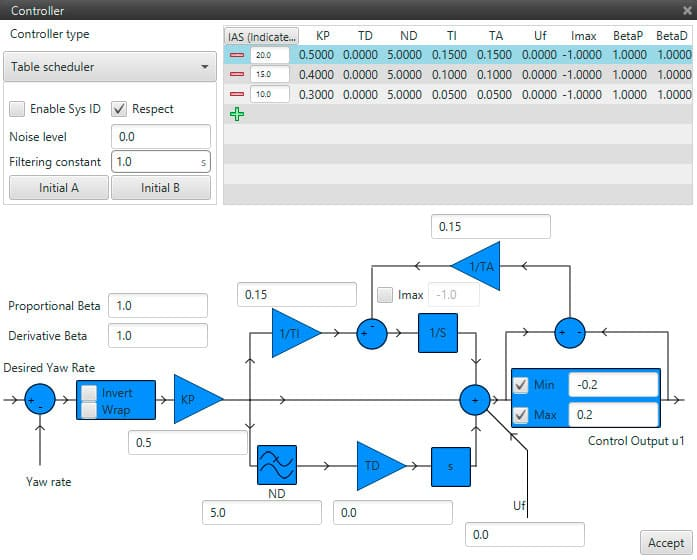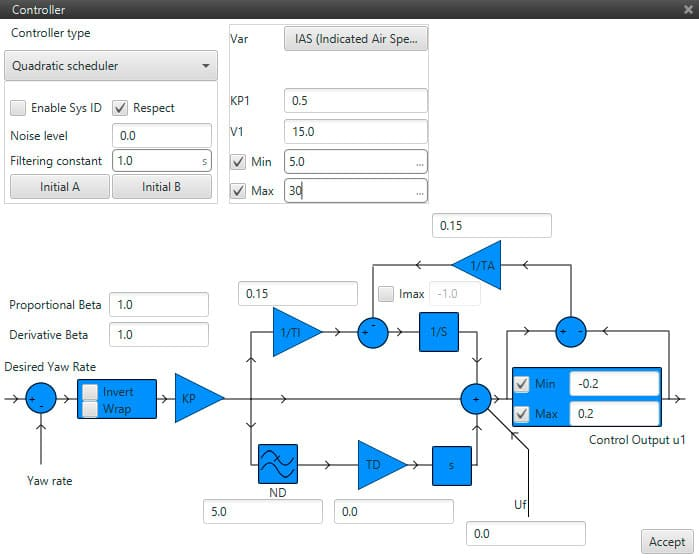# Gain Scheduling¶

This aproach uses a group of linear controllers for different operation points. Each controller adjusts its gains automatically according to scheduling variables.

Veronte incorporates 4 approaches for this type of control:

• Table Scheduler: gain scheduling controller with variation of the parameters according to a table interpolation. In this option, the values of the PID controller vary according to a variable that is selected when this option is marked. For example, if the IAS is selected as the scheduling parameter, depending on the velocity of the aircraft the parameters of the PID will change.Table Scheduler controller

• Inverse Scheduler: gain scheduling controller with variation of the parameters according to the selected variable using inverse proportionality.

• Proportional Scheduler: gain scheduling controller with variation of the parameters according to the selected variable using direct proportionality.

• Quadratic Scheduler: gain scheduling controller with variation of the parameters according to the selected variable using quadratic proportionality.

For the last three approaches, the only gain that changes according to a certain variable is the proportional one. Now there is not a table interpolation but a mathematical expression, that can be inverse (KP1/V1=cte), proportional (KP1 * V1=cte) and quadratic (KP1 *V1^2=cte). The nominal point given by the expressions will remain fixed. Therefore, the proportional gain will change when V1 varies in order to mantain the same value.Scheduler table

In the previous expressions, shall be clear that limits are applied to the variable (V) and not to the gain (Kp).

The following figure shows the quadratic case, where the proportional gain changes according to the IAS, having as nominal point (KP1 and V1) 0.5 and 15 m/s respectively.

The values Min and Max are used to establish a limit of the scheduler. Below and above those limits, the system works as a conventional PID with the gain indicated in KP1.